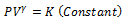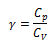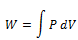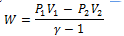• Process in which no heat enters or leaves a system is called an adiabatic process
• For every adiabatic process Q=0
• Prevention of heat flow can be accomplished by the surrounding system with a thick layer of heat insulating material like cork, asbestos etc.
• The flow of heat requires finite time so if a process is performed very quickly then the process will be practically adiabatic.
• On applying first law to adiabatic process we get

ΔU=U2 – U1= – ΔW               (adiabatic process)

• In adiabatic process change in internal energy of a system is equal in magnitude to the work by the system.

If work is done on the system contracts i.e. ΔW is negative then and internal energy of system increases by an amount equal to the work done on it and temperature of system increases.

ΔU = ΔW

1. If work is done by the system i.e., ΔW is negative

ΔU = – ΔW

here internal energy of systems decreases resulting a drop in temperature.

## Work done in an adiabatic process:

• For an adiabatic process of ideal gas equation we haveWhere γ is the ratio of specific heat (ordinary or molar) at constant pressure and at constant volume• Suppose in an adiabatic process pressure and volume of a sample of gas changes from (P1, V1) to (P2, V2) then we haveThus,• Work done by gas in this process iswhere limits of integration goes from V1 to V2

Putting for P = K/Vγ, and integrating we get,• In an adiabatic process if W>0 i.e., work is done by the gas then T2< T1
• If work is done on the gas (W<0) then T2 > T1 i.e., temperature of gas rises.

# Isochoric process:

• In an isochoric process volume of the system remain unchanged throughout i.e. ΔV = O.
• When volume does not change any work is done; ΔW = 0 and therefore from first law

U2 – U1 = ΔU =ΔQ

• All the heat given to the system has been used to increase the internal energy of the system.

# Isobaric Process:

• A process taking place at constant pressure is called isobaric process.
• Work done in the isobaric process is where the pressure is kept constant.

W = P (V2 – V1) = μ R (T2-T1)

• Here in this process the amount of heat given to the system is partly used in increasing temperature and partly used in doing work.